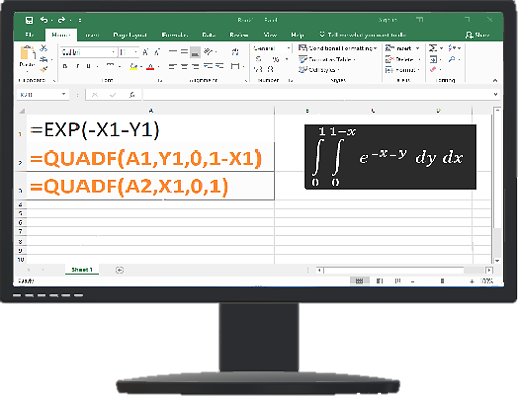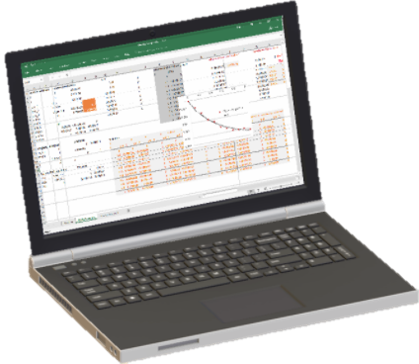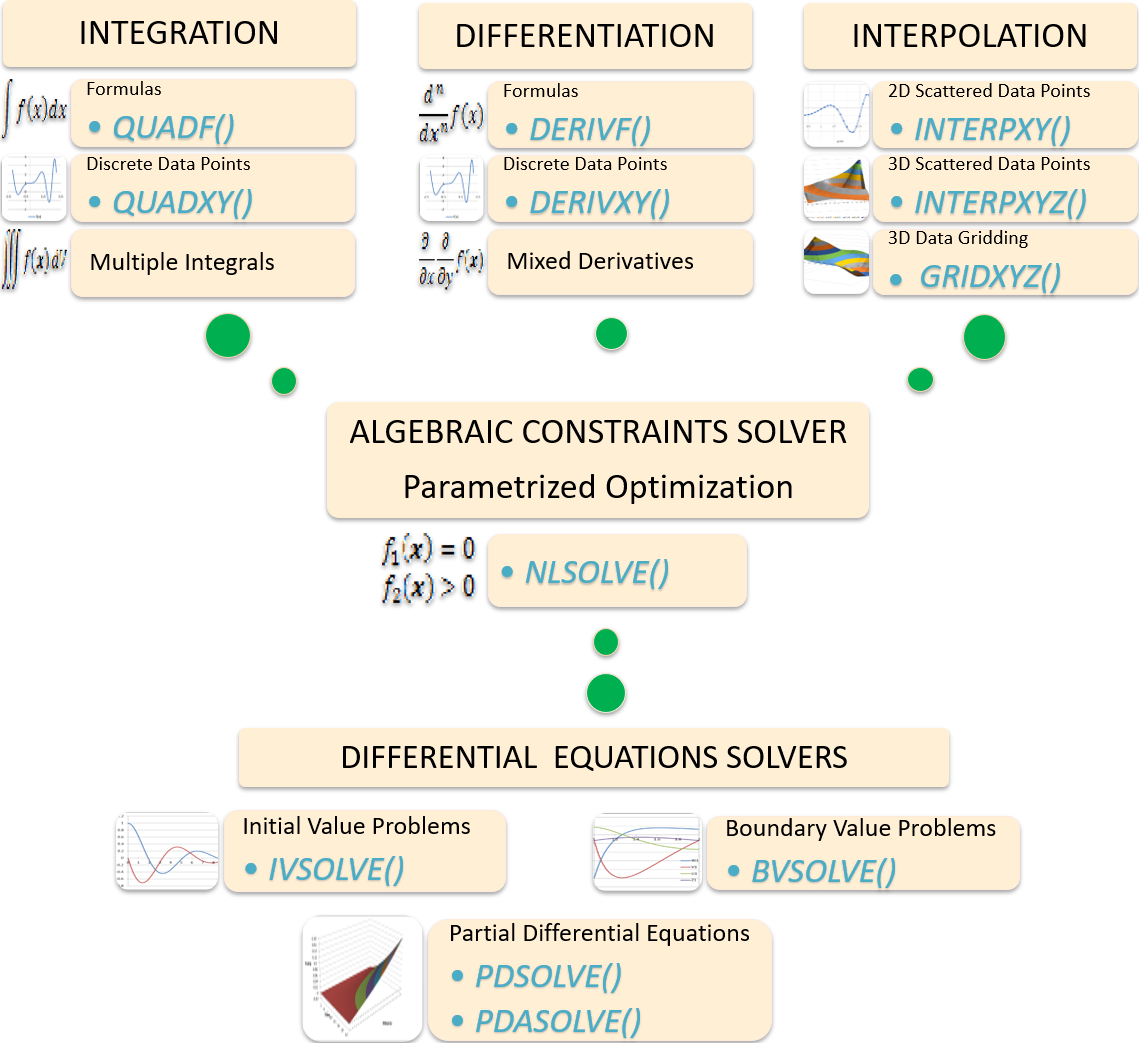#ExceLabTM 3.0Excel Calculus Powerhouse Add-in

## Solve complex calculus problems with simple formulas

• Expand Excel native math function with a super set of powerful solvers.

• Use the solvers in standard formulas exactly like native math functions.

• No coding. No learning curves. Just basic Excel spreadsheet skills.

• Lightweight add in with superfast 20-sec installation and you are up and running.

• State of the art proven algorithms that work with superior performance.

• Share your results workbook easily with your co-workers.

• Evaluate free to your satisfaction.

• Get prompt support and answers to questions from our experienced team.## What our users say

See full reviews

".. the ability to share models and results in a highly familiar platform..with near-zero learning-curve in using the program, have compelled me to break from the past and put in the effort to move our models to ExceLab."
"It is easy to learn and support provided by the admin staff is very nice. If anyone working on multi PDEs equation problems, just try this one you will love this solver"
"I found excel-works derivative functions quite useful. I was particularly pleased with the prompt response for assistance when I required some technical issues to be resolved....."
What can you solve with ExceLab

ExceLab is a unique add-in which extends Excel native math functions with a super set of powerful calculus functions. You use these functions in standard or array formulas just like native functions to solve elementary and advanced calculus problems including:

The functions offer you state of the art algorithms to effortlessly and accurately solve calculus problems with just basic spreadsheet skills and no programming. Navigate the Tabs above for quick examples.

## Compute numerical integrals

• Use QUADF to integrate formulas and user defined VBA functions

• Use QUADXY to integrate (x,y) data points

• Nest QUADF to compute multiple integrals of any order

• Highly accurate adaptive algorithms

• Finite and infinite limits

• Singular integrands

### Quick Example

#### Solution

 A 1 =LN(X1)/SQRT(X1) 2 =QUADF(A1,X1,0,1)
 A 1 #NUM! 2 -4

## Compute numerical derivatives

• Use DERIVF to compute derivatives of formulas and VBA functions

• Use DERIVXY to compute derivatives from (x,y) data points

• Nest DERIVF to compute partial derivatives of any order

• First and higher-order derivatives

• Highly-accurate adaptive algorithm

### Quick Example

$f\left(x\right)=xsin\left({x}^{2}\right)+1$

#### Solution

 A 1 =X1*SIN(X1^2)+1 2 =DERIVF(A1,X1,PI())
 A 1 1 2 -18.248596

## Interpolate 2D and 3D data points

• Use INTERPXY to interpolate scattered 2D (x,y) points

• Use INTERPXYZ to interpolate scattered 3D (x,y,z) points

• Map your scattered (x,y) and (x,y,z) onto uniform grids and plot the curve or surface in Excel

• Best known Natural Neighbour Algorithm

### Quick Example: gridding and plotting 3D Scattered points

 A B C 1 x y z 2 0.124162 0.011109 0.124162 3 0.468730 0.229740 0.747031 .. ↓ 51 0.222274 0.049164 0.635259
 E F G .. P 1 0 0.1 → 1 2 0 =INTERPXYZ(A2:B51, z, grid_x, grid_y) 3 0.1 .. ↓ 12 1Highlight and insert Excel Surface plot

## Find exact or best solution

• Use NLSOLVE to solve a system of nonlinear equations

• Find least error solution to coupled equations and inequalities constraints

• Find Roots of non-linear equations

• Proven Levenberg-Marquardt Algorithm

### Quick Example

${10}^{4}{x}_{1}{x}_{2}-1=0$ ${e}^{-{x}_{1}}+{e}^{-{x}_{2}}-1.0001=0$

#### Solution

 A X 1 =10^4*X1*X2-1 1 2 =EXP(-X1)+EXP(-X2)-1.0001 1
 A B 4 =NLSOLVE(A1:A2,X1:X2) 5
 A B 4 X1 9.10614674 5 X2 1.09816E-05

## Solve ordinary differential equations

• Use IVSOLVE to solve an initial value problem ODE system

• Use BVSOLVE to solve a boundary value problem ODE system

• Highly-staple highly-accurate fully-implicit adaptive algorithms

• Formatted output ready for plotting in Excel

### Quick initial value ode example

$\frac{dv}{dt}=-2\zeta \omega v-{\omega }^{2}x$ t ∈ [0, 12]

#### Solution

 A B C D 2 t w 1 3 x 1 zeta 0.25 4 v 0 6 dx/dt =v 7 dv/dt =-2*zeta*w*v-w^2*x
 I J K 1 =IVSOLVE(B6:B7,(t,x,v),{0,12}) .. 32

## Solve partial differential equations

• Use PDSOLVE or to solve partial differential equations

• Use PDASOLVE to solve partial differential algebraic equations

• Robust Method of Lines with adaptive time step

• Multiple regions with discontinuous properties

• General boundary conditions

• Flexible output formats for plotting transient and snapshot views

### Quick Example: heat equation

t ∈ [0,1], x ∈ [0,1]

#### Solution

 Left Bc Right Bc A B C D 1 t k 1 2 x 3 u =IF(x=0,100,0) 4 ux 5 uxx 6 du/dt =k*uxx =u-100 =ux
 A B C D 8 =PDSOLVE(B6,B1:B5,C6,D6,{0,1},{0,1}) .. 30

## Solve dynamical optimization and parameter estimation problems

### Numerous Applications

• Optimize your model parameters for best fit with experimental data

• Estimate differential equations parameters

• Maximize or minimize an objective formula subject to dynamic constraints with Excel Solver

See related journal publication Rapid Modeling and Parameter Estimation of Partial Differential Algebraic Equations by a Functional Spreadsheet Paradigm.Math. Comput. Appl. 2018, 23, 39.

### Quick Example: compute unknown integral limits

$f\left(x,a,b\right)=\underset{a}{\overset{b}{\int }}1-{x}^{2}+b\cdot dx$

Find $a,b$ such that

$f\left(x,a,b\right)=8.333333$

### Solution

 A B 1 a 0 2 b 1 3 integrand =1-x1^2+b 4 integral =QUADF(B3,X1,a,b) 5 constraint =B4-8.333333
 A B 7 =NLSOLVE(B5,(a,b)) 8
 A B 7 a -2 8 b 3

## Solve optimal control problems

#### FInd u(t) and x(t) to maximize (or minimize) a cost index J

$J=H\left(\mathbit{x}\left(T\right),T\right)+\underset{0}{\overset{T}{\int }}G\left(\mathbit{x}\left(t\right),\mathbit{u}\left(t\right),t\right)dt$

#### Subject to

$\frac{d\mathbit{x}}{dt}=f\left(\mathbit{x}\left(t\right),\mathbit{u}\left(t\right),t\right)$ $\mathbit{x}\left(0\right)={\mathbit{x}}_{0}$ $\mathbit{x}\left(T\right)={\mathbit{x}}_{T}$ $\left|\mathbit{u}\left(t\right)\right|\le {\mathbit{u}}_{max}$
• Obtain rapid solutions with minimal effort and simple formulas. No programming.

• Novel structured direct solution procedure combining Excel Solver with the calculus functions.

• Method applicable to general (non standard) forms of optimal control problems .

• Remarkable convergence and performance illustrated by several examples.

• Consistent and sometime better results than complex methods.

Why should you work with us

### Our product is engineered with over two-decades experience in computational calculus. ExceLab add-in is based on an innovative patented technology that represents a breakthrough in the utilization of the spreadsheet application with natural extension of native math functions to calculus. ExceLab functions are unique and have no parallels in the history of the spreadsheet application since its inception in the seventies.ExceLab the simpler alternative to heavy-weight tools

• We stand behind our product which stands on its own merits.

• We help you with fast and effective support from our expert staff.

• We deliver timely fixes to your issues.

• We listen to your needs. Get involved in shaping the next release with features that matter to you.

• lightweight efficient library with one click installation and you are up and running.

• State of the art proven algorithms that work. Best default settings yet you have full control with optional arguments.

• Get rapid solutions to elementary and advanced calculus problems effortlessly with simple formulas in familiar Excel. Leave tedious coding and expensive complex tools behind

• Flexible licensing plans that suit your needs.

### We invite you to explore the solved problems in the manual and evaluate freely to experience the difference for yourself.

Teach your class with ExceLab
• Excel is virtually on every computer. Very easy to learn the basics and use.

• Help your students spend their time solving problems, not figuring out how to use complex tools.

• Intutive yet powerful differential equations solvers and integrated optimization.

• Simplified effective approach to optimal control problems for Engineering and Social Studies.

### Contact us for practical arrangements that suit your requirements.

ExceLab functions at a glanceQuestion or Comment? Email us:
support @ excel-works.comExceLab: Transforming Excel into a Calculus Power House
ExceLab functions and methods are protected by USA Patents 9286286, 9892108, 10114812 and pending.
© 2015-2019, ExcelWorks LLC
Boston, USA